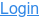Close# Level Up Your Unit Tests: How to Turn a JUnit Test into a Fuzz Test

Unit tests are indispensable to check and prove that our code functions properly. But in unit testing, we only test the scenarios that we are aware of. However, there are scenarios unknown to us that lead to security vulnerabilities or performance problems. To address these scenarios, you can add fuzz tests in order to effectively find security, reliability, and even logic bugs in your code.

CI Fuzz offers a JUnit integration which makes writing fuzz tests as easy as writing normal unit tests. This means that if you already have unit tests for your code, you turn those into fuzz tests with minor effort. In this post, we will walk you through how you can do this and shed light on key aspects to keep in mind to guarantee that our fuzz tests are effective.

For this example, we will start with a unit test that tests our SomeScheme class that implements encode and decode methods. A Unit test might look like this:

``    @Test    public void existingUnitTest() {         String input1 = "foo";         String encoded1 = SomeScheme.encode(input1);         assertEquals(input1, SomeScheme.decode(encoded1));         String input2 = "bar";         String encoded2 = SomeScheme.encode(input2);         assertEquals(input2, SomeScheme.decode(encoded2));         String input3 = "baz";         String encoded3 = SomeScheme.encode(input3);         assertEquals(input3, SomeScheme.decode(encoded3));    }``

This represents a classic example of unit testing where we check that our code works properly for fixed predefined inputs. Here, we test that decoding an encoded string results in the original input string for three concrete inputs. This test does not tell us how our code would react to other inputs. Are there special cases where decoding an encoded input would lead to a different text than the original one? This would indicate a logic bug in our code. To answer these questions, we can use fuzzing which can effectively explore program behavior and trigger interesting corner cases.

## Creating a Fuzz Test

CI Fuzz offers the @FuzzTest annotation that you can use to create a parameterized test with parameters generated automatically by CI Fuzz. We can use the same logic we use in our unit test but instead of providing concrete manually, we provide the input generated by CI Fuzz.

`` @FuzzTest void fuzzTest(FuzzedDataProvider data) {      String input = data.consumeRemainingAsString();      String encoded = SomeScheme.encode(input);      assertEquals(input, SomeScheme.decode(encoded)); }``

CI Fuzz will then execute a method in a loop and in each iteration provide new inputs `data` that maximize code coverage and trigger interesting behavior in your application. This is very effective in triggering corner cases that you might have overseen.

If you can formulate properties or invariants that should be true for all inputs to your code, then you can use fuzzing to find counterexamples where these properties do not hold. When turning a unit test into a fuzz test, you need to adjust the assertions with ones that represent invariants that should be true for all inputs.

Keep in mind that the fuzz tests became shorter than the corresponding unit test since we don’t have to specify concrete inputs but only use the fuzzer-generated inputs.

## Conclusion

We’ve shown a simple approach for converting JUnit unit tests into fuzz tests. This approach can be applied to any Java project using JUnit.